# AP Physics C: Mechanics Practice Test 8

### Test Information11 questions15 minutes

1. A bicycle slows down with an acceleration whose magnitude increases linearly with time. Which of the following velocity–time graphs could represent the motion of the bicycle?

2.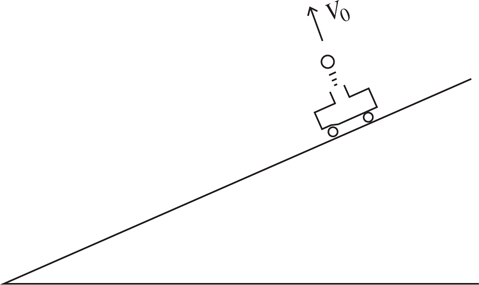A cart is sliding down a low friction incline. A device on the cart launches a ball, forcing the ball perpendicular to the incline, as shown above. Air resistance is negligible. Where will the ball land relative to the cart, and why?

3. The quantity “jerk,” j, is defined as the time derivative of an object’s acceleration,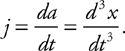What is the physical meaning of the area under a graph of jerk vs. time?

4. A particle moves along the x-axis with a position given by the equation x(t) = 5 + 3t, where x is in meters, and t is in seconds. The positive direction is east. Which of the following statements about the particle is FALSE.

5.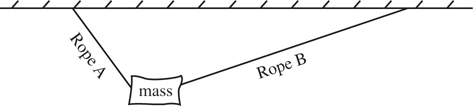A mass hangs from two ropes at unequal angles, as shown above. Which of the following makes correct comparisons of the horizontal and vertical components of the tension in each rope?

6.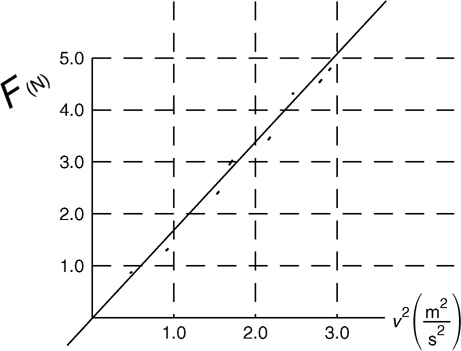The force of air resistance F on a mass is found to obey the equation F = bv2, where v is the speed of the mass, for the range of speeds investigated in an experiment. A graph of F vs. v2 is shown above. What is the value of b?

7. A box sits on an inclined plane without sliding. As the angle of the plane (measured from the horizontal) increases, the normal force

8. Which of the following conditions are necessary for an object to be in static equilibrium?

I. The vector sum of all torques on the object must equal zero.

II. The vector sum of all forces on the object must equal zero.

III. The sum of the object’s potential and kinetic energies must be zero.

9.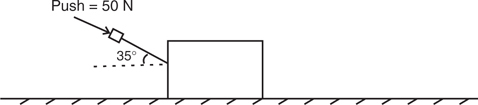A student pushes a big 16-kg box across the floor at constant speed. He pushes with a force of 50 N angled 35° from the horizontal, as shown in the diagram above. If the student pulls rather than pushes the box at the same angle, while maintaining a constant speed, what will happen to the force of friction?

10. Consider a system consisting only of the Earth and a bowling ball, which moves upward in a parabola above Earth’s surface. The downward force of Earth’s gravity on the ball, and the upward force of the ball’s gravity on the Earth, form a Newton’s third law force pair. Which of the following statements about the ball is correct?

11.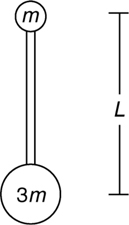A mass m is attached to a mass 3m by a rigid bar of negligible mass and length L. Initially, the smaller mass is located directly above the larger mass, as shown above. How much work is necessary to flip the rod 180° so that the larger mass is directly above the smaller mass?